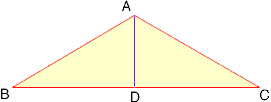I have to find the area of an isoceles triangle with one angle side of 30 degrees, and length of base 5. Could you please help me solve this problem? Thank you. Hi, I drew a diagram of an isosceles triangle with a 30o angle at B, and hence also at C. Th base BC has length 5.D is the midpoint of BC so angle BDA is a right angle. Hence triangle ABD is a 30-60-90 triangle. You can use trigonometry to find the length of AD but I remember that in a 30-60-90 triangle the sides are in the proportions 1:2:root(3). Thus BD is root(3) times as long as AD. |BD| = 2.5 and hence |AD| = 2.5/root(3). |AD| is the height of the triangle ABC and hence you can find its area. Cheers, Penny Go to Math Central To return to the previous page use your browser's back button.